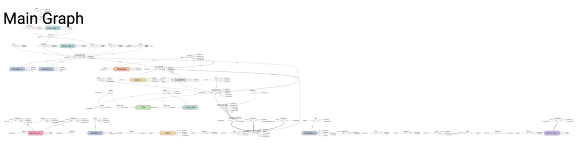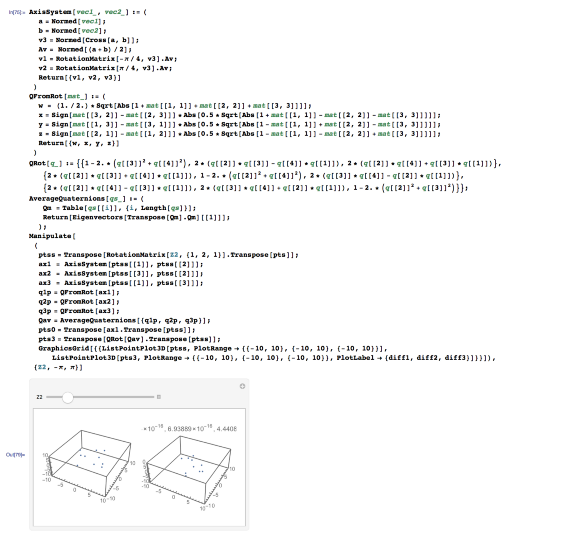At atomsandbits.ai we implement some seriously large formulas in TensorFlow. If we just went from LaTeX to tf. we wouldn’t be able to do it. Here’s a list of tricks and tools we use, applied to the problem of averaging rotations. Come for the tf. stay for the hypersphere.

The tensormol0.2 model chemistry reproduces a huge swath of chemistry (37 elements), which is in some sense a large fraction of our world. It’s a big ole’ formula for some geometry:How does one use TensorFlow effectively to get something complicated done? It’s not easy. I thought I’d write up an example a little simpler than modeling all of chemistry. How about averaging rotations/axis systems? Simple right? Well interesting story… The math is mostly due to Hamilton (~1843), however it wasn’t until the advent of computer graphics in 1985 that people even bothered to work out how to interpolate between rotations perfectly.

#Rotation & Quaternions

The rotational algebra of our world is a beautiful bedeviling thing. The reason is that although rotations act on a three dimensional space, when embedded in three dimensions, rotations are not smooth or unique. When represented with Euler angles or matrices, every rotation has multiple representations. Change the order of rotations and you also change the endpoint (rotations are non-commutative) Traveling smoothly along some paths of rotations using a three dimensional embedding, suddenly the third degree of freedom can become inaccessible (the phenomenon of “Gimbal lock”). If you try to define an average or interpolated point-of-view in a naive way (axes=> angles => interpolated angles) you will find gibberish zero axes, and jerky non-smooth behavior.

The rotational algebra of our world is a beautiful bedeviling thing. The reason is that although rotations act on a three dimensional space, when embedded in three dimensions, rotations are not smooth or unique. When represented with Euler angles or matrices, every rotation has multiple representations. Change the order of rotations and you also change the endpoint (rotations are non-commutative) Traveling smoothly along some paths of rotations using a three dimensional embedding, suddenly the third degree of freedom can become inaccessible (the phenomenon of “Gimbal lock”). If you try to define an average or interpolated point-of-view in a naive way (axes=> angles => interpolated angles) you will find gibberish zero axes, and jerky non-smooth behavior.

To have smooth topology rotations must be embedded within a four-dimensional hypersphere, so we can forgive your brain. In this space a rotation is a 4-dimensional point, a quaternion, whose components can be thought of as the angle and 3 axis components of the rotation. Given a 3x3 rotation matrix Q, one can parameterize a quaternion (w,x,y,z)Given any set of orthogonal axes (rows of Q), Euler’s theorem guarantees an axis-angle rotation which can map the natural xyz axes back and forth into the new frame. The formula above yields the natural 4-d form of that rotation.

Now suppose you have two, three or four systems of axes (ax_1, ax_2, ax_3). For example you want to look at the sun then the moon, or you want to fit 4 pretty objects in your field of vision, or define invariant axes for a cloud of points (the reason we use this math in TensorMol). Can you simply average the quaternion components q_av = (ax_1+ax_2+ax_3)/3? Sadly no… You can immediately understand why if you imagine averaging rotations around opposite axis vectors as an owl might when spinning his head. The “good, smooth” quaternions keep to the surface of the 4-d hypersphere (a curvy subset of 4d-euclidean space). To interpolate lines on that sphere, you can use SLERP. To average multiple quaternions we must construct the 4x4 matrix which is the outer product of the list of quaternions (Nx4) with itself, weighted if desired:

$$Q = q_1, q_2, ...$$ $$M = (w\cdot Q)^t Q$$

The largest eigenvector of this matrix is the desired average quaternion.

# Implementing complex math in tf.

Again, my goal is to get rotationally invariant axes for a set of points which smooth, differentiable and local. I will walk through my whole implementation of this in tf. Step 1- Don’t use tf. Write a simple test of your formulas in a notebook like math interface (mathematica, ipython/sage). Verify everything is working when you use all the fancy library routines tf. doesn’t have (eigenvectors etc.). Here’s what that looks like using mathematica.Those fancy manipulate sliders are a nice way to get tangible faith that the point cloud is rotationally invariant when transformed using an averaged axis system depending on points in the cloud. It remains for us to do this same thing in tf. We’re ready for step 2:

Step 2: Power up a jupyter notebook and translate into tensorflow. It’s simply faster to avoid integration with the rest of the package while trying to debug some involved set of mathematical manipulations like this. The idea is to make a minimal notebook that also tests all the possible edge cases which could cause numerical instability. Here for example is some code showing that the mapping between axis systems and quaternions works invertibly:

Style notes about the tf. code given above:

• Each routine can be easily compared with the mathematica output, to quickly debug.
• In general it is best to choose an order of dimensions for your tensor which goes (least often contracted,…,most often contracted), because several helpful tf. functions assume the first dimension of a tensor is the batch dimension.
• tf.sqrt, and 1/(tensor) are both unstable operations in tf. They are unstable in a tricky way, because the implied chain-rule derivative graph (coming from tf.gradients(… op…, var) will still often evaluate NaN’s even when it appears that the arguments to the routine would always be in the well-behaved domain. One must make liberal use of tf.clip_by_value() , tf.where(), and infinitesimals to ensure both the routine and routine’s derivatives are well-behaved. Safe_norm is a good example.

# Epilogue

So is all this serious rotational mathematics only good for defining axis systems for atomic positions. No! Facebook AI-Research and collaborators from the EPFL published a nice use of quaternions for skeletal motion planning last week